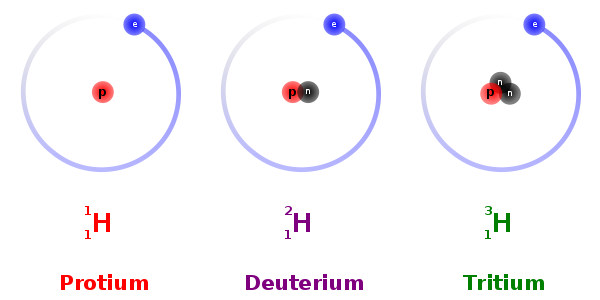# Nuclei - Online Test

Q1. What is the percentage of the mass of an atom concentrated in the nucleus?
Explaination / Solution:

A proton and a neutron have roughly 2000 times the mass of an electron. The numbers of an electron in a stable atom (not isotope) is equal to the electrons. So for most atoms, the nucleus will hold roughly 4000 times the mass of its electron cloud. Thus,
Q2. What is the unit of mass when measured on the atomic scale?
Explaination / Solution:

The unified atomic mass unit (u) is defined as one twelfth of the mass of an unbound neutral atom of carbon-12 in its nuclear and electronic ground state and at rest.

Q3. Atomic mass unit (u) is defined as ________ of the mass of the carbon () atom.
Explaination / Solution:

For standardization, a specific atomic nucleus (carbon-12) had to be chosen because the average mass of a nucleon depends on the count of the nucleons in the atomic nucleus due to mass defect. This is also why the mass of a proton or neutron by itself is more than (and not equal to) 1 u.

Q4. A free neutron decays into
Explaination / Solution:

Free neutrons are unstable. A free neutron () decays into a proton (), an electron (), and an electron antineutrino (). This phenomenon is called beta decay.

Q5. Relation between atomic number (Z), neutron number (N) and mass number (A) is
Explaination / Solution:

mass number (A) = total number of nucleons = number of protons + number of nutrons = atomic number (Z) + neutron number (N).

Q6. Nuclear species or nuclides are shown by the notation ____________ where X is the chemical symbol of the species.
Explaination / Solution:

Atomic mass number (total number of nucleons) and the atomic number (number of protons) of the species are given by A and Z, respectively.

Q7.  Contains
Explaination / Solution:

Number of protons = Atomic number = 79 Number of neutrons = Atomic mass number - atomic number = 197-79 = 118.

Q8. The nuclei of isotopes of a given element contain the same number of
Explaination / Solution:

Isotopes are variants of a particular chemical element which differ in neutron number. All isotopes of a given element have the same number of protons in each atom.Q9. The nuclei of isotopes of a given element differ from each other in their number of
Explaination / Solution:

Isotopes are variants of a particular chemical element which differ in neutron number. All isotopes of a given element have the same number of protons in each atom.Q10. All nuclides with same mass number A are called
Explaination / Solution:

These are the elements having same atomic number but different mass number. They have the same atomic number because the number of protons inside their nuclei remains the same. The difference in their mass number is due to the difference in their number of neutrons.